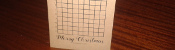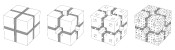mscroggs.co.uk
mscroggs.co.uksubscribe

# Blog

2017-12-18
Just like last year, TD and I spent some time in November this year designing a puzzle Christmas card for Chalkdust.
The card looks boring at first glance, but contains 10 puzzles. Converting the answers to base 3, writing them in the boxes on the front, then colouring the 1s black and 2s orange will reveal a Christmassy picture.
If you want to try the card yourself, you can download this pdf. Alternatively, you can find the puzzles below and type the answers in the boxes. The answers will be automatically converted to base 3 and coloured...
 # Answer (base 10) Answer (base 3) 1 0 0 0 0 0 0 0 2 0 0 0 0 0 0 0 3 0 0 0 0 0 0 0 4 0 0 0 0 0 0 0 5 0 0 0 0 0 0 0 6 0 0 0 0 0 0 0 7 0 0 0 0 0 0 0 8 0 0 0 0 0 0 0 9 0 0 0 0 0 0 0 10 0 0 0 0 0 0 0
1. In a book with 116 pages, what do the page numbers of the middle two pages add up to?
2. What is the largest number that cannot be written in the form $$14n+29m$$, where $$n$$ and $$m$$ are non-negative integers?
3. How many factors does the number $$2^6\times3^{12}\times5^2$$ have?
4. How many squares (of any size) are there in a $$15\times14$$ grid of squares?
5. You take a number and make a second number by removing the units digit. The sum of these two numbers is 1103. What was your first number?
6. What is the only three-digit number that is equal to a square number multiplied by the reverse of the same square number? (The reverse cannot start with 0.)
7. What is the largest three-digit number that is equal to a number multiplied by the reverse of the same number? (The reverse cannot start with 0.)
8. What is the mean of the answers to questions 6, 7 and 8?
9. How many numbers are there between 0 and 100,000 that do not contain the digits 0, 1, 2, 3, 4, 5, or 6?
10. What is the lowest common multiple of 52 and 1066?

### Similar postsChristmas card 2016Christmas card 2018Christmas (2018) is overChristmas (2018) is coming!

Comments in green were written by me. Comments in blue were not written by me.
2019-01-04
@Jose: There is a mistake in your answer: 243 (0100000) is the number of numbers between 10,000 and 100,000 that do not contain the digits 0, 1, 2, 3, 4, 5, or 6.
2019-01-04
Thanks for the puzzle!
Is it possible that the question 9 is no correct?
I get a penguin with perfect simetrie except at answer 9 : 0100000 that breaks the simetry.
Is it correct or a mistake in my answer?
Thx
2018-01-01
@C: look up something called Frobenius numbers. This problem's equivalent to finding the Frobenius number for 14 and 29.
2017-12-28
I can solve #2 with code, but is there a tidy maths way to solve it directly?
2017-12-23
My efforts were flightless.

Allowed HTML tags: <br> <a> <small> <b> <i> <s> <sup> <sub> <u> <spoiler> <ul> <ol> <li>
To prove you are not a spam bot, please type "z" then "e" then "r" then "o" in the box below (case sensitive):
2016-12-20
Last week, I posted about the Christmas card I designed on the Chalkdust blog.
The card looks boring at first glance, but contains 12 puzzles. Converting the answers to base 3, writing them in the boxes on the front, then colouring the 1s green and 2s red will reveal a Christmassy picture.
If you want to try the card yourself, you can download this pdf. Alternatively, you can find the puzzles below and type the answers in the boxes. The answers will be automatically converted to base 3 and coloured...
 # Answer (base 10) Answer (base 3) 1 0 0 0 0 0 0 0 0 0 2 0 0 0 0 0 0 0 0 0 3 0 0 0 0 0 0 0 0 0 4 0 0 0 0 0 0 0 0 0 5 0 0 0 0 0 0 0 0 0 6 0 0 0 0 0 0 0 0 0 7 0 0 0 0 0 0 0 0 0 8 0 0 0 0 0 0 0 0 0 9 0 0 0 0 0 0 0 0 0 10 0 0 0 0 0 0 0 0 0 11 0 0 0 0 0 0 0 0 0 12 0 0 0 0 0 0 0 0 0
1. The square number larger than 1 whose square root is equal to the sum of its digits.
2. The smallest square number whose factors add up to a different square number.
3. The largest number that cannot be written in the form $$23n+17m$$, where $$n$$ and $$m$$ are positive integers (or 0).
4. Write down a three-digit number whose digits are decreasing. Write down the reverse of this number and find the difference. Add this difference to its reverse. What is the result?
5. The number of numbers between 0 and 10,000,000 that do not contain the digits 0, 1, 2, 3, 4, 5 or 6.
6. The lowest common multiple of 57 and 249.
7. The sum of all the odd numbers between 0 and 66.
8. One less than four times the 40th triangle number.
9. The number of factors of the number $$2^{756}$$×$$3^{12}$$.
10. In a book with 13,204 pages, what do the page numbers of the middle two pages add up to?
11. The number of off-diagonal elements in a 27×27 matrix.
12. The largest number, $$k$$, such that $$27k/(27+k)$$ is an integer.

### Similar postsChristmas card 2017Christmas card 2018Christmas (2018) is overChristmas (2018) is coming!

Comments in green were written by me. Comments in blue were not written by me.
2016-12-20
@Matthew: Thank you for the prompt response! It makes sense now and perhaps I should have read a little closer!
2016-12-20
@Dan Whitman: Find the difference between the original number and the reverse of the original. Call this difference $$a$$. Next add $$a$$ to the reverse of $$a$$...
2016-12-20
In number 4 what are we to take the difference between? Do you mean the difference between the original number and its reverse? If so when you add the difference back to the reverse you simply get the original number, which is ambiguous. I am not sure what you are asking us to do here.